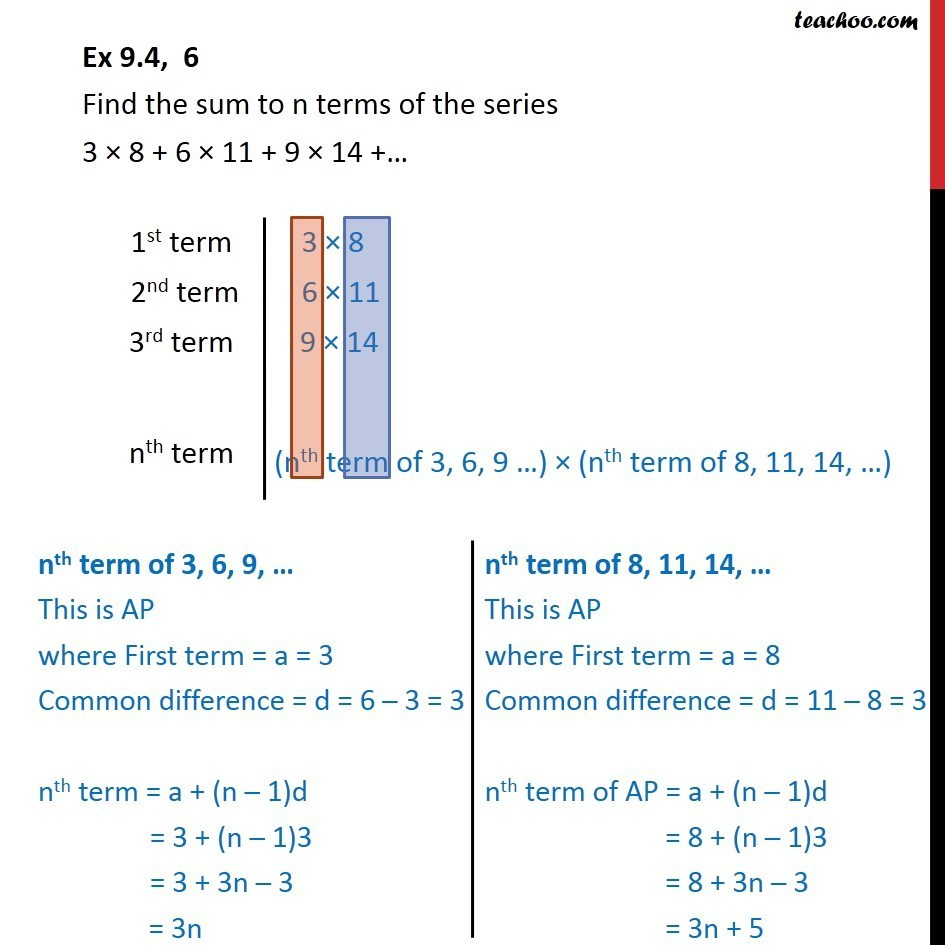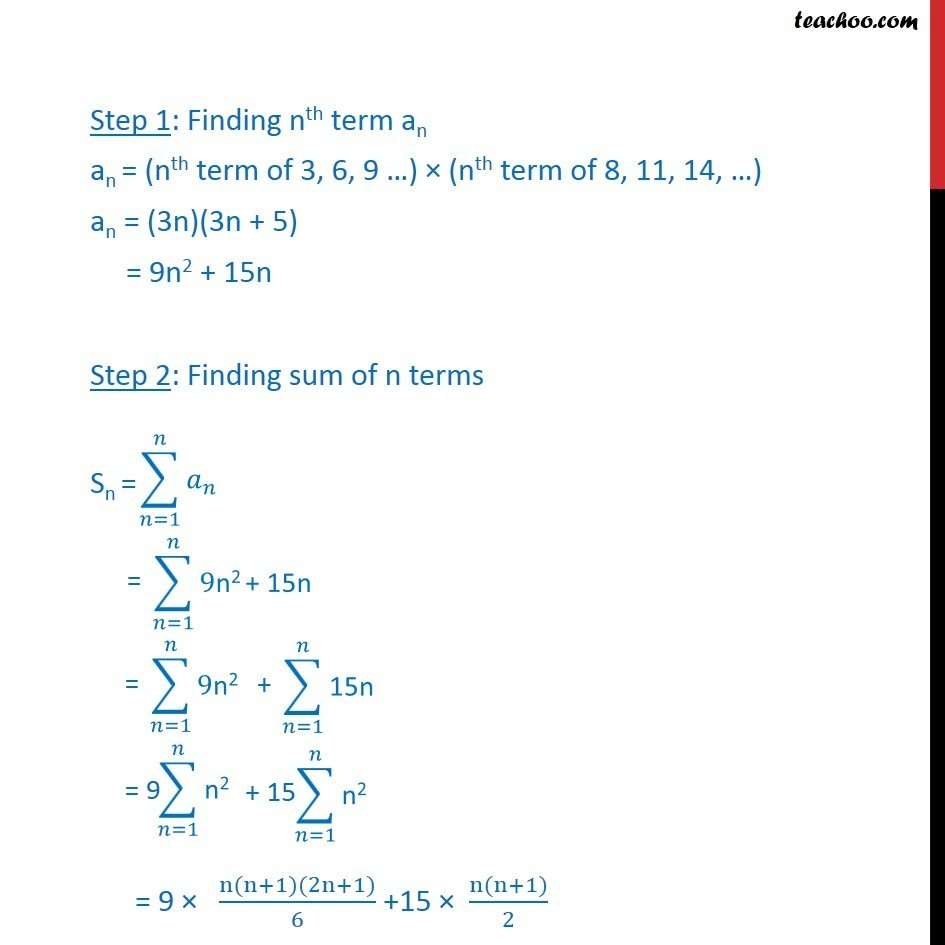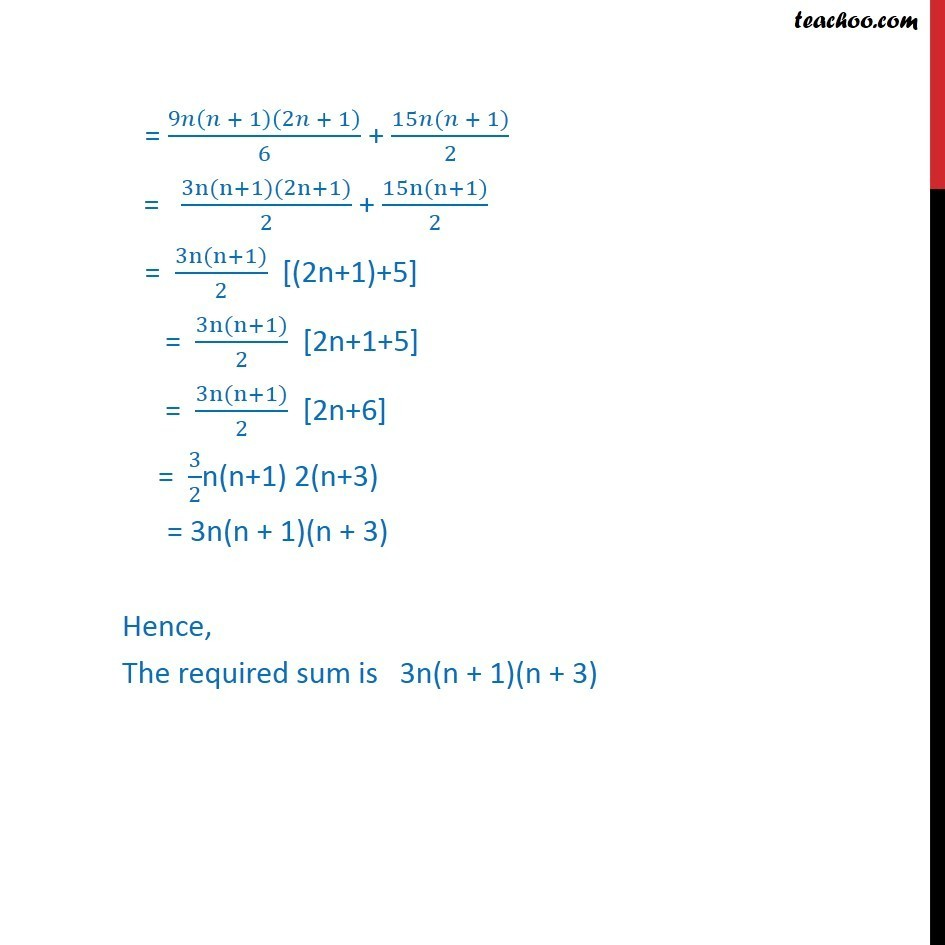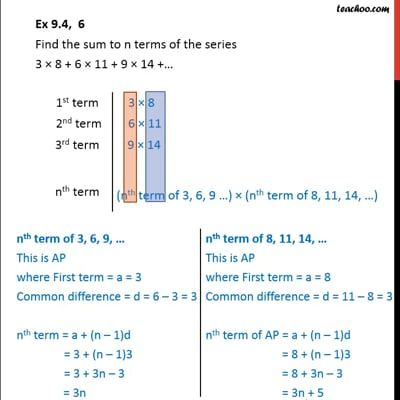Ex 9.4

Chapter 9 Class 11 Sequences and Series
Serial order wiseThis video is only available for Teachoo black users

Get live Maths 1-on-1 Classs - Class 6 to 12

### Transcript

Ex 9.4, 6 Find the sum to n terms of the series 3 8 + 6 11 + 9 14 + Step 1: Finding nth term an an = (nth term of 3, 6, 9 ) (nth term of 8, 11, 14, ) an = (3n)(3n + 5) = 9n2 + 15n Step 2: Finding sum of n terms = 9 (n(n+1)(2n+1))/6 +15 (n(n+1))/2 = 9 ( + 1)(2 + 1)/6 + (15 ( + 1))/2 = (3n(n+1)(2n+1))/2 + (15n(n+1))/2 = (3n(n+1))/2 [(2n+1)+5] = (3n(n+1))/2 [2n+1+5] = (3n(n+1))/2 [2n+6] = 3/2n(n+1) 2(n+3) = 3n(n + 1)(n + 3) Hence, The required sum is 3n(n + 1)(n + 3)